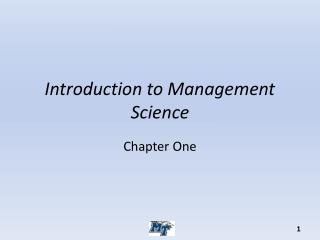DownloadDownload PresentationIntroduction to Management Science

Introduction to Management Science

Télécharger la présentationIntroduction to Management Science

- - - - - - - - - - - - - - - - - - - - - - - - - - - E N D - - - - - - - - - - - - - - - - - - - - - - - - - - -
Presentation Transcript

1. Introduction to Management Science Chapter One

2. What is Management Science? • Management Science is a “discipline” that attempts to aid managerial decision making by applying a scientific approach to managerial problems that involve quantitative factors.

3. Why Study Management Science? • Gain an appreciation for the relevance and power of MS. • Learn to recognize when MS can (and cannot)be fruitfully Applied • Learn how to apply the major techniques of MS to analyze a variety or managerial problems. • Develop an understanding of how to interpret the results of a MS study.

4. The Nature of Management Science • MS is a Discipline • Current Roots in 1940’s and 50’s • Operations Research, OR, MS, MS/OR • Simplex algorithm, Danzig (1947) • MS aids managerial decision making • MS uses a scientific approach

5. Management Science Uses a Scientific Approach • Define the problem and gather data. • Formulate a model (typically a mathematic model) to represent the problem. • Develop a computer-based procedure for deriving solutions to the problem from the model. • Test the model and refine it as needed. • Apply the model to analyze the problem and develop recommendations for management. • Help to implement the team’s recommendations that are adopted by management

6. Special Products Break-Even Analysis • The Special Products Company produces expensive and unusual gifts. • The latest new-product proposal is a limited edition grandfather clock. • Data: • If they go ahead with this product, a fixed cost of \$50,000 is incurred. • The variable cost is \$400 per clock produced. • Each clock sold would generate \$900 in revenue. • A sales forecast will be obtained. Question: Should they produce the clocks, and if so, how many?

7. Expressing the Problem Mathematically • Decision variable: • Q = Number of grandfather clocks to produce • Costs: • Fixed Cost = \$50,000 (if Q > 0) • Variable Cost = \$400 Q • Total Cost = • 0, if Q = 0 • \$50,000 + \$400 Q, if Q > 0 • Profit: • Profit = Total revenue – Total cost • Profit = 0, if Q = 0 • Profit = \$900Q – (\$50,000 + \$400Q) = –\$50,000 + \$500Q, if Q > 0